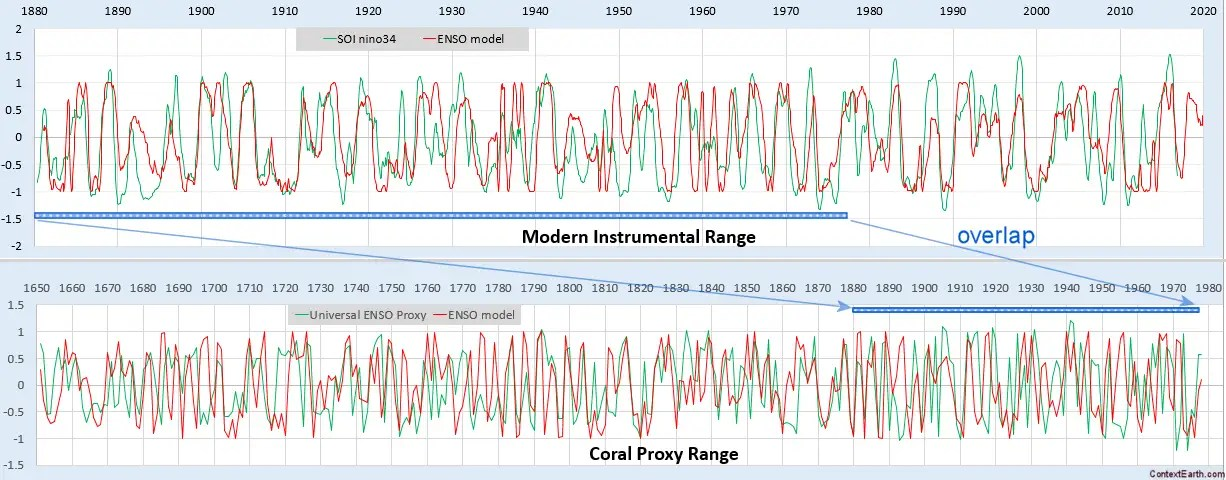# The ENSO Forcing Potential – Cheaper, Faster, and Better

Following up on the last post on the ENSO forcing, this note elaborates on the math.  The tidal gravitational forcing function used follows an inverse power-law dependence, where a(t) is the anomalistic lunar distance and d(t) is the draconic or nodal perturbation to the distance.$F(t) propto frac{1}{(R_0 + a(t) + d(t))^2}'$

Note the prime indicating that the forcing applied is the derivative of the conventional inverse squared Newtonian attraction. This generates an inverse cubic formulation corresponding to the consensus analysis describing a differential tidal force:$F(t) propto -frac{a'(t)+d'(t)}{(R_0 + a(t) + d(t))^3}$

For a combination of monthly and fortnightly sinusoidal terms for a(t) and d(t) (suitably modified for nonlinear nodal and perigean corrections due to the synodic/tropical cycle)   the search routine rapidly converges to an optimal ENSO fit.  It does this more quickly than the harmonic analysis, which requires at least double the unknowns for the additional higher-order factors needed to capture the tidally forced response waveform. One of the keys is to collect the chain rule terms a'(t) and d'(t) in the numerator; without these, the necessary mixed terms which multiply the anomalistic and draconic signals do not emerge strongly.

As before, a strictly biennial modulation needs to be applied to this forcing to capture the measured ENSO dynamics — this is a period-doubling pattern observed in hydrodynamic systems with a strong fundamental (in this case annual) and is climatologically explained by a persistent year-to-year regenerative feedback in the SLP and SST anomalies.

Here is the model fit for training from 1880-1980, with the extrapolated test region post-1980 showing a good correlation.The geophysics is now canonically formulated, providing (1) a simpler and more concise expression, leading to (2) a more efficient computational solution, (3) less possibility of over-fitting, and (4) ultimately generating a much better correlation. Alternatively, stated in modeling terms, the resultant information metric is improved by reducing the complexity and improving the correlation — the vaunted  cheaper, faster, and better solution. Or, in other words: get the physics right, and all else follows.

# ENSO Proxy Validation

This is a straightforward validation of the ENSO model presented at last December’s AGU.

What I did was use the modern instrumental record of ENSO — the NINO34 data set — as a training interval, and then tested across the historical coral proxy record — the UEP data set.The correlation coefficient in the out-of-band region of 1650 to 1880 is excellent, considering that only two RHS lunar periods (draconic and anomalistic month) are used for forcing. As a matter of fact, trying to get any kind of agreement with the UEP using an arbitrary set of sine waves is problematic as the time-series appears nearly chaotic and thus requires may Fourier components to fit. With the ENSO model in place, the alignment with the data is automatic. It predicts the strong El Nino in 1877-1878 and then nearly everything before that.

# Shortest Training Fit for ENSO

This is remarkable. Using the spreadsheet linked in the last post, the figure below is a model of ENSO derived completely by a training fit over the interval 1900 to 1920, using the Nino3.4 data series and applying the precisely phased Draconic and Anomalistic long-period tidal cycles.Fig. 1 : The ENSO model in red. The blue BG region is used for training of the lunar tidal amplitudes against the Nino3.4 data in green. That data is square root compacted to convert it to an equivalent velocity.

Not much more to say. There is a major disturbance starting in the mid-1980’s, but that is known from a Takens embedding analysis described in the first paper in this post.